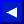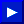When evaluating a number for truth or falsehood, the computer assumes the number is true as long as its value isn't 0. When evaluating a comparison, it assigns a value of -I if the result is true, while false has a value of 0. In binary format, -1 is all 1's and 0 is all 0's. Therefore, when ANDing true/false evaluations, the result will be true if any bits in the result are true.

EXAMPLES of Using AND with True/False Evaluations:
```   50 IF X=7 AND W=3 THEN GOTO 10: REM ONLY TRUE IF BOTH X=7
AND W=3 ARE TRUE
60 IF A AND Q=7 THEN GOTO 10: REM TRUE IF A IS NON-ZERO
AND Q=7 IS TRUE
```

### ASC

TYPE: Function-Numeric
FORMAT: ASC(<string>)

Action: ASC will return a number from 0 to 255 which corresponds to the Commodore ASCII value of the first character in the string. The table of Commodore ASCII values is shown in Appendix C.

EXAMPLES OF ASC Function:
```   10 PRINT ASC("Z")
20 X = ASC("ZEBRA")
30 J = ASC(J\$)
```

If there are no characters in the string, an ?ILLEGAL QUANTITY error results. In the third example above, if J\$="", the ASC function will not work. The GET and GET# statement read a CHR\$(0) as a null string. To eliminate this problem, you should add a CHR\$(0) to the end of the string as shown below.

EXAMPLE of ASC Function Avoiding ILLEGAL QUANTITY ERROR:
```   30 J = ASC(J\$ + CHR\$(0))
```This page has been created by Sami Rautiainen. Read the small print. Last updated December 22, 2002.